# Overwrite Values

Overwrite submatrix or subdiagonal of input

## Library

• Math Functions / Matrices and Linear Algebra / Matrix Operations

`dspmtrx3`

• Signal Management / Indexing

`dspindex`

•## Description

The Overwrite Values block overwrites a contiguous submatrix or subdiagonal of an input matrix. You can provide the overwriting values by typing them in a block parameter, or through an additional input port, which is useful for providing overwriting values that change at each time step.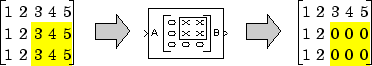The block accepts scalars, vectors and matrices. The output always has the same size as the original input signal, not necessarily the same size as the signal containing the overwriting values. The input(s) and output of this block must have the same data type.

### Specifying the Overwriting Values

The Source of overwriting value(s) parameter determines how you must provide the overwriting values, and has the following settings.

• `Specify via dialog` — You must provide the overwriting value(s) in the Overwrite with parameter. The block uses the same overwriting values to overwrite the specified portion of the input at each time step. To learn how to specify valid overwriting values, see Valid Overwriting Values.

• `Second input port` — You must provide overwriting values through a second block input port, `V`. Use this setting to provide different overwriting values at each time step. The output inherits its size and rate from the input signal, not the overwriting values.

The rate at which you provide the overwriting values through input port `V` must match the rate at which the block receives each input matrix at input port `A`. In other words, the input signals must have the same Simulink® sample time.

### Valid Overwriting Values

The overwriting values can be a single constant, vector, or matrix, depending on the portion of the input you are overwriting, regardless of whether you provide the overwriting values through an input port or by providing them in the Overwrite with parameter.

Valid Overwriting Values

Portion of Input to OverwriteValid Overwriting ValuesExample

A single element in the input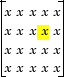Any constant value, v

v` = 9`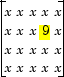A length-k portion of the diagonal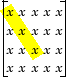Any length-k column or row vector, v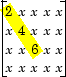A length-k portion of a row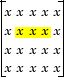Any length-k row vector, v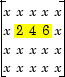A length-k portion of a column

`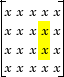`

Any length-k column vector, v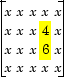An m-by-n submatrix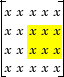Any m-by-n matrix, v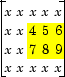This block supports Simulink virtual buses.

## Parameters

Note

Only some of the following parameters are visible in the dialog box at any one time.

Overwrite

Determines whether to overwrite a specified submatrix or a specified portion of the diagonal.

Source of overwriting value(s)

Determines where you must provide the overwriting values: either through an input port, or by providing them in the Overwrite with parameter. For more information, see Specifying the Overwriting Values.

Overwrite with

The value(s) with which to overwrite the specified portion of the input matrix. Enabled only when Source of overwriting value(s) is set to ```Specify via dialog```. To learn how to specify valid overwriting values, see Valid Overwriting Values.

Row span

The range of input rows to be overwritten. Options are ```All rows```, `One row`, or ```Range of rows```. For descriptions of these options, see Parameters.

Row/Starting row

The input row that is the first row of the submatrix that the block overwrites. For a description of the options for the Row and Starting row parameters, see Settings for Row, Column, Starting Row, and Starting Column Parameters. Row is enabled when Row span is set to `One row`, and Starting row when Row span is set to `Range of rows`.

Row index/Starting row index

Index of the input row that is the first row of the submatrix that the block overwrites. See how to use these parameters in Settings for Row, Column, Starting Row, and Starting Column Parameters. Row index is enabled when Row is set to `Index`, and Starting row index when Starting row is set to `Index`.

Row offset/Starting row offset

The offset of the input row that is the first row of the submatrix that the block overwrites. See how to use these parameters in Settings for Row, Column, Starting Row, and Starting Column Parameters. Row offset is enabled when Row is set to `Offset from middle `or `Offset from last`, and Starting row offset is enabled when Starting row is set to `Offset from middle` or ```Offset from last```.

Ending row

The input row that is the last row of the submatrix that the block overwrites. For a description of this parameter's options, see Settings for Ending Row and Ending Column Parameters. This parameter is enabled when Row span is set to `Range of rows`, and Starting row is set to any option but `Last`.

Ending row index

Index of the input row that is the last row of the submatrix that the block overwrites. See how to use this parameter in Settings for Ending Row and Ending Column Parameters. Enabled when Ending row is set to `Index`.

Ending row offset

The offset of the input row that is the last row of the submatrix that the block overwrites. See how to use this parameter in Settings for Ending Row and Ending Column Parameters. Enabled when Ending row is set to `Offset from middle` or ```Offset from last```.

Column span

The range of input columns to be overwritten. Options are ```All columns```, `One column`, or ```Range of columns```. For descriptions of the analogous row options, see Parameters.

Column/Starting column

The input column that is the first column of the submatrix that the block overwrites. For a description of the options for the Column and Starting column parameters, see Settings for Row, Column, Starting Row, and Starting Column Parameters. Column is enabled when Column span is set to `One column`, and Starting column when Column span is set to ```Range of columns```.

Column index/Starting column index

Index of the input column that is the first column of the submatrix that the block overwrites. See how to use these parameters in Settings for Row, Column, Starting Row, and Starting Column Parameters. Column index is enabled when Column is set to `Index`, and Starting column index when Starting column is set to `Index`.

Column offset/Starting column offset

The offset of the input column that is the first column of the submatrix that the block overwrites. See how to use these parameters in Settings for Row, Column, Starting Row, and Starting Column Parameters. Column offset is enabled when Column is set to `Offset from middle` or `Offset from last`, and Starting column offset is enabled when Starting column is set to ```Offset from middle``` or `Offset from last`.

Ending column

The input column that is the last column of the submatrix that the block overwrites. For a description of this parameter's options, see Settings for Ending Row and Ending Column Parameters. This parameter is enabled when Column span is set to `Range of columns`, and Starting column is set to any option but `Last`.

Ending column index

Index of the input column that is the last column of the submatrix that the block overwrites. See how to use this parameter in Settings for Ending Row and Ending Column Parameters. This parameter is enabled when Ending column is set to `Index`.

Ending column offset

The offset of the input column that is the last column of the submatrix that the block overwrites. See how to use this parameter in Settings for Ending Row and Ending Column Parameters. This parameter is enabled when Ending column is set to `Offset from middle` or `Offset from last`.

Diagonal span

The range of diagonal elements to be overwritten. Options are ```All elements```, `One element`, or ```Range of elements```. For descriptions of these options, see Overwriting a Subdiagonal.

Element/Starting element

The input diagonal element that is the first element in the subdiagonal that the block overwrites. For a description of the options for the Element and Starting element parameters, see Element and Starting Element Parameters. Element is enabled when Element span is set to `One element`, and Starting element when Element span is set to `Range of elements`.

Element index/Starting element index

Index of the input diagonal element that is the first element of the subdiagonal that the block overwrites. See how to use these parameters in Element and Starting Element Parameters. Element index is enabled when Element is set to `Index`, and Starting element index when Starting element is set to `Index`.

Element offset/Starting element offset

The offset of the input diagonal element that is the first element of the subdiagonal that the block overwrites. See how to use these parameters in Element and Starting Element Parameters. Element offset is enabled when Element is set to ```Offset from middle``` or `Offset from last`, and Starting element offset is enabled when Starting element is set to ```Offset from middle``` or `Offset from last`.

Ending element

The input diagonal element that is the last element of the subdiagonal that the block overwrites. For a description of this parameter's options, see Ending Element Parameters. This parameter is enabled when Element span is set to `Range of elements`, and Starting element is set to any option but `Last`.

Ending element index

Index of the input diagonal element that is the last element of the subdiagonal that the block overwrites. See how to use this parameter in Ending Element Parameters. This parameter is enabled when Ending element is set to `Index`.

Ending element offset

The offset of the input diagonal element that is the last element of the subdiagonal that the block overwrites. See how to use this parameter in Ending Element Parameters. This parameter is enabled when Ending element is set to ```Offset from middle``` or `Offset from last`.

## Examples

### Overwriting a Submatrix

To overwrite a submatrix, follow these steps:

1. Set the Overwrite parameter to `Submatrix`.

2. Specify the overwriting values as described in Specifying the Overwriting Values.

3. Specify which rows and columns of the input matrix are contained in the submatrix that you want to overwrite by setting the Row span parameter to one of the following options and the Column span to the analogous column-related options:

• `All rows` — The submatrix contains all rows of the input matrix.

• `One row` — The submatrix contains only one row of the input matrix, which you must specify in the Row parameter, as described in the following table.

• `Range of rows` — The submatrix contains one or more rows of the input, which you must specify in the Starting Row and Ending row parameters, as described in the following tables.

4. When you set Row span to ```One row``` or `Range of rows`, you need to further specify the row(s) contained in the submatrix by setting the Row or Starting row and Ending row parameters. Likewise, when you set Column span to `One column` or `Range of columns`, you must further specify the column(s) contained in the submatrix by setting the Column or Starting column and Ending column parameters. For descriptions of the settings for these parameters, see the following tables.

Settings for Row, Column, Starting Row, and Starting Column Parameters

Settings for Specifying the Submatrix's First Row or ColumnFirst Row of Submatrix
(Only row for Row span = One row)
First Column of Submatrix
(Only row for Row span = One row)

`First`

First row of the input

First column of the input

`Index`

Input row specified in the Row index parameter

Input column specified in the Column index parameter

`Offset from last`

Input row with the index
`M` - ```rowOffset ```where `M` is the number of input rows, and `rowOffset` is the value of the Row offset or Starting row offset parameter

Input column with the index
`N` - ```colOffset ```where `N` is the number of input columns, and `colOffset` is the value of the Column offset or Starting column offset parameter

`Last`

Last row of the input

Last column of the input

`Offset from middle`

Input row with the index
```floor(M/2 + 1 - rowOffset) ```where `M` is the number of input rows, and `rowOffset` is the value of the Row offset or Starting row offset parameter

Input column with the index `floor(N/2 + 1 - rowOffset)` where `N` is the number of input columns, and `colOffset` is the value of the or Column offset or Starting column offset parameter

`Middle`

Input row with the index
```floor(M/2 + 1) ```where `M` is the number of input rows

Input columns with the index `floor(N/2 + 1) ` where `N` is the number of input columns

Settings for Ending Row and Ending Column Parameters

Settings for Specifying the Submatrix's Last Row or ColumnLast Row of SubmatrixLast Column of Submatrix

`Index`

Input row specified in the Ending row index parameter

Input column specified in the Ending column index parameter

`Offset from last`

Input row with the index
`M` - ```rowOffset ```where `M` is the number of input rows, and `rowOffset` is the value of the Ending row offset parameter

Input column with the index
`N` - ```colOffset ```where `N` is the number of input columns, and `colOffset` is the value of the Ending column offset parameter

`Last`

Last row of the input

Last column of the input

`Offset from middle`

Input row with the index
```floor(M/2 + 1 - rowOffset) ```where `M` is the number of input rows, and `rowOffset` is the value of the Ending row offset parameter

Input column with the index
```floor(N/2 + 1 - rowOffset) ``` where `N` is the number of input columns, and `colOffset` is the value of the Ending column offset parameter

`Middle`

Input row with the index
```floor(M/2 + 1) ```where `M` is the number of input rows

Input columns with the index `floor(N/2 + 1)` where `N` is the number of input columns

For example, to overwrite the lower-right 2-by-3 submatrix of a 3-by-5 input matrix with all zeros, enter the following set of parameters:

• Overwrite = `Submatrix`

• Source of overwriting value(s) = ```Specify via dialog```

• Overwrite with = `0`

• Row span = `Range of rows`

• Starting row = `Index`

• Starting row index = `2`

• Ending row = `Last`

• Column span = `Range of columns`

• Starting column = `Offset from last`

• Starting column offset = `2`

• Ending column = `Last`

The following figure shows the block with the above settings overwriting a portion of a 3-by-5 input matrix.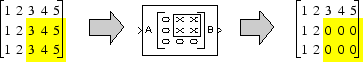There are often several possible parameter combinations that select the same submatrix from the input. For example, instead of specifying `Last` for Ending column, you could select the same submatrix by specifying

• Ending column = `Index`

• Ending column index = `5`

### Overwriting a Subdiagonal

To overwrite a subdiagonal, follow these steps:

1. Set the Overwrite parameter to `Diagonal`.

2. Specify the overwriting values as described in Specifying the Overwriting Values.

3. Specify the subdiagonal that you want to overwrite by setting the Diagonal span parameter to one of the following options:

• `All elements` — Overwrite the entire input diagonal.

• `One element` — Overwrite one element in the diagonal, which you must specify in the Element parameter (described below).

• `Range of elements` — Overwrite a portion of the input diagonal, which you must specify in the Starting element and Ending element parameters, as described in the following table.

4. When you set Diagonal span to ```One element``` or `Range of elements`, you need to further specify which diagonal element(s) to overwrite by setting the Element or Starting element and Ending element parameters. See the following tables.

Element and Starting Element Parameters

Settings for Element and Starting Element ParametersFirst Element in Subdiagonal
(Only element when Diagonal span = One element)

`First`

Diagonal element in first row of the input

`Index`

kth diagonal element, where k is the value of the Element index or Starting element index parameter

`Offset from last`

Diagonal element in the row with the index
`M` - ```offset ```where `M` is the number of input rows, and `offset` is the value of the Element offset or Starting element offset parameter

`Last`

Diagonal element in the last row of the input

`Offset from middle`

Diagonal element in the input row with the index
```floor(M/2 + 1 - offset) ```where `M` is the number of input rows, and `offset` is the value of the Element offset or Starting element offset parameter

`Middle`

Diagonal element in the input row with the index
```floor(M/2 + 1) ```where `M` is the number of input rows

Ending Element Parameters

Settings for Ending Element ParameterLast Element in Subdiagonal

`Index`

kth diagonal element, where k is the value of the Ending element index parameter

`Offset from last`

Diagonal element in the row with the index
`M` - ```offset ```where `M` is the number of input rows, and `offset` is the value of the Ending element offset parameter

`Last`

Diagonal element in the last row of the input

`Offset from middle`

Diagonal element in the input row with the index
```floor(M/2 + 1 - offset) ```where `M` is the number of input rows, and `offset` is the value of the Ending element offset parameter

`Middle`

Diagonal element in the input row with the index
```floor(M/2 + 1) ```where `M` is the number of input rows

## Supported Data Types

The input(s) and output of this block must have the same data type.

PortSupported Data Types

A

• Double-precision floating point

• Single-precision floating point

• Fixed point (signed and unsigned)

• Boolean

• 8-, 16-, and 32-bit signed integers

• 8-, 16-, and 32-bit unsigned integers

V

• Double-precision floating point

• Single-precision floating point

• Fixed point (signed and unsigned)

• Boolean

• 8-, 16-, and 32-bit signed integers

• 8-, 16-, and 32-bit unsigned integers

B

• Double-precision floating point

• Single-precision floating point

• Fixed point (signed and unsigned)

• Boolean

• 8-, 16-, and 32-bit signed integers

• 8-, 16-, and 32-bit unsigned integers

 Reshape (Simulink) Simulink Selector (Simulink) Simulink Submatrix DSP System Toolbox Variable Selector DSP System Toolbox `reshape` MATLAB

## Extended Capabilities

### Fixed-Point ConversionDesign and simulate fixed-point systems using Fixed-Point Designer™.

Introduced before R2006a

## Support평가판 신청